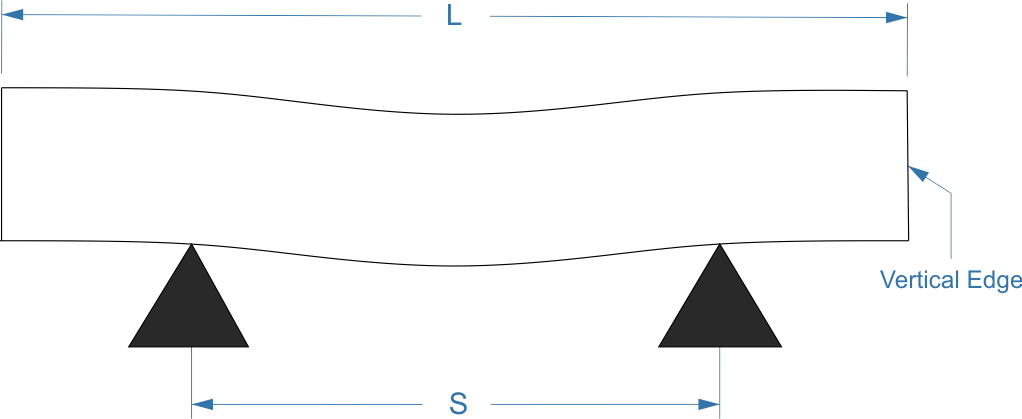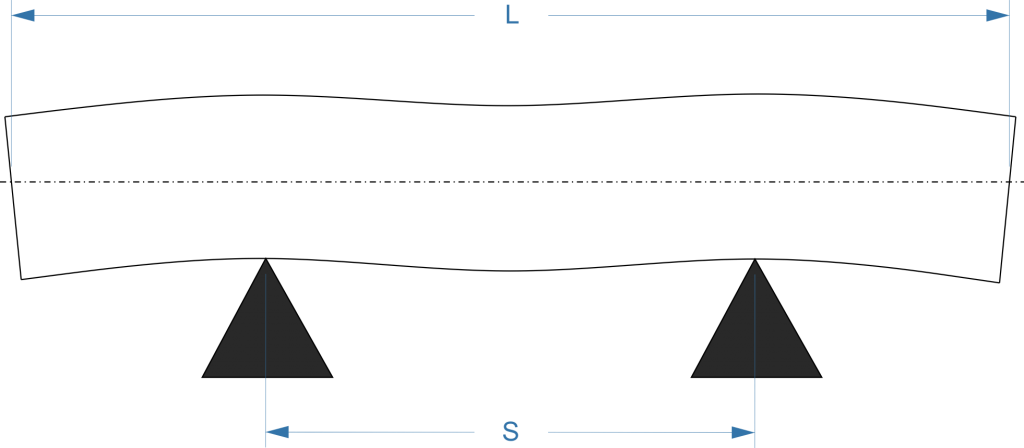When simply supported at finite number of points, a beam will bend under its own weight. The shape it takes depends on the spacing between the supports and the cross-sectional and micro-structural uniformity of the beam.

## Airy Points

Sir George Biddell Airy  showed that the ends of the bar can be made completely horizontal when a beam or bar is supported at a special set of points. These points are known as airy points and following equation describes their location in a system with two supports:

$$s =\frac{L}{\sqrt{3}}$$

where,

s : Distance separating the supports
L : Length of the barSince $$\frac{1}{\sqrt{3}}=0.577$$, the above relationship is sometimes abbreviated as:

$$s =0.577L$$

The relationship was derived [PDF] using the Euler-Bernouilli beam bending equation and it can be used provided the following set of assumptions is applicable to the beam:

1. It  is homogeneous and isotropic
2. Has uniform cross-sectional area
3. It is linear-elastic

Unless these assumptions are true, the calculated airy point might not coincide with the actual one.

## Bessel Points

In case we are trying to minimise the maximum extent the member deflects from the centre line, then the bessel point should be used for support instead. Bessel Points can be calculated using the following formula.

$$s =0.559L$$### Application: Support of Length Standards

Sufficient care has to be taken when length standards, such as gauge blocks, are used for comparison purposes. Length standards of small size (< 3 inches) are quasi-non affected by any change in length no matter the method of support. However, longer standards can experience appreciable changes in length due to gravity. For example if the support method is vertical a reduction in length will occur because of the compression due to gravity. The change in length can be found using:

$${\Delta}{L} = \frac{DL^2}{2E}$$

where,

ΔL : Change in Length
D   : Density
L   : Original Length
E   : Young’s Modulus

However, if the standard is supported horizontally at its airy points, the effect of gravity on length can be almost neglected. This method of support can also be used for end standards.